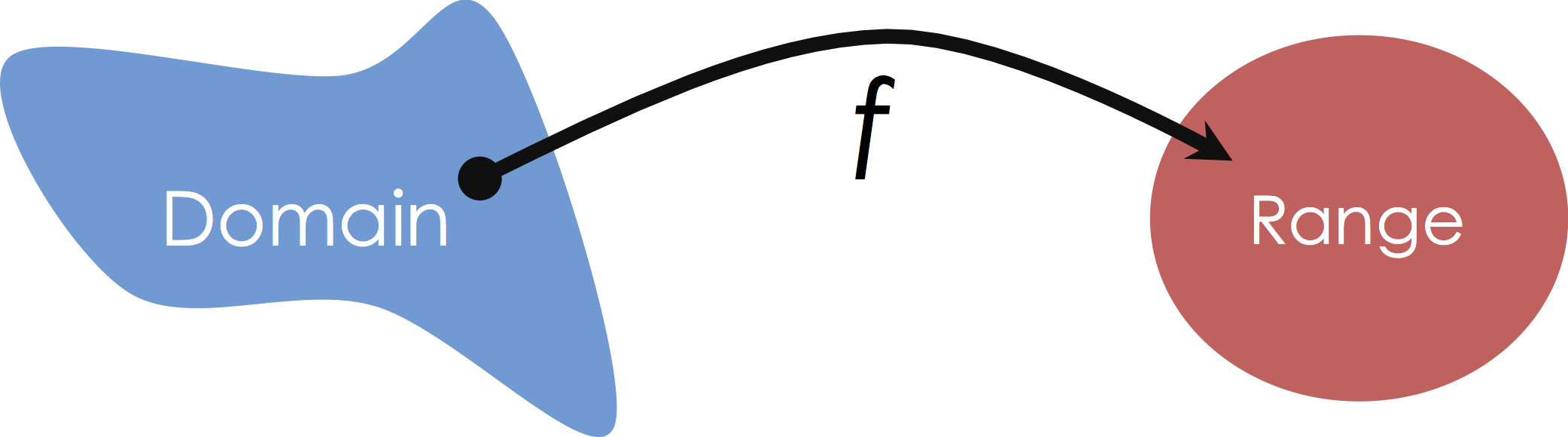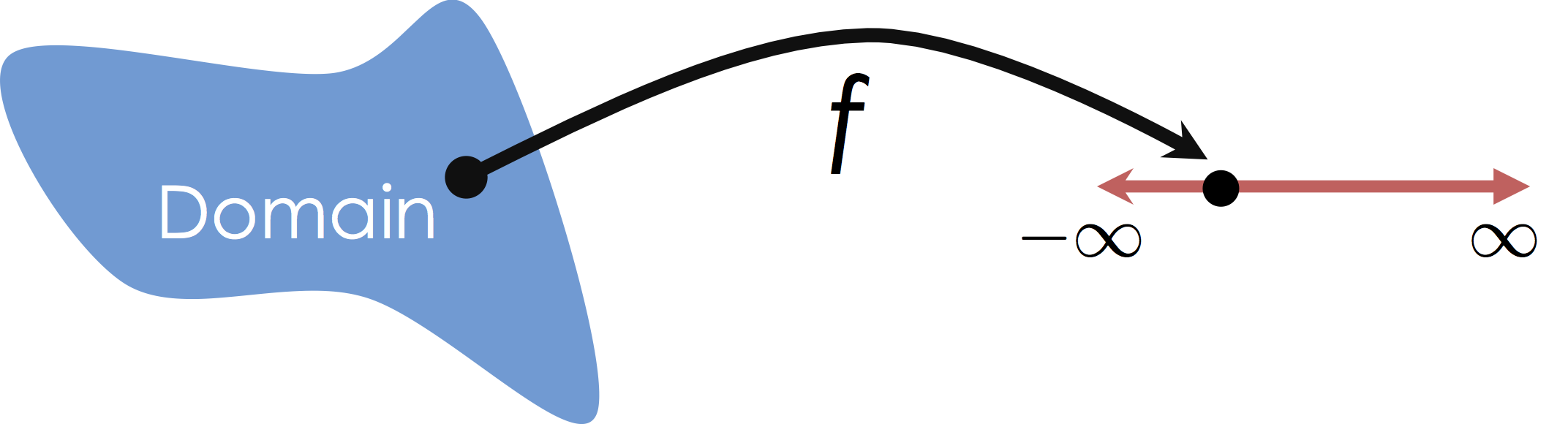# Lecture 14, Part 2 – Data 100, Fall 2020¶

by Joseph Gonzalez (Spring 2020)

## Feature Engineering¶

In this notebook we will explore a key part of data science, feature engineering: the process of transforming the representation of model inputs to enable better model approximation. Feature engineering enables you to:

1. encode non-numeric features to be used as inputs to common numeric models
2. capture domain knowledge (e.g., the perceived loudness or sound is the log of the intensity)
3. transform complex relationships into simple linear relationships

## Mapping from Domain to Range¶

In the past few lectures we have been exploring various models for regression. These are models from some domain to a continuous quantity.

So far we have been interested in modeling relationships from some numerical domain to a continuous quantitative range:In this class we will focus on Multiple Regression in which we consider mappings from potentially high-dimensional input spaces onto the real line (i.e., $y \in \mathbb{R}$):It is worth noting that this is distinct from Multivariate Regression in which we are predicting multiple (confusing?) response values (e.g., $y \in \mathbb{R}^q$).

### Standard Imports¶

As usual, we will import a standard set of functions.

In :
import numpy as np
import pandas as pd

In :
import plotly.offline as py
import plotly.express as px
import plotly.graph_objects as go
import plotly.figure_factory as ff
cf.set_config_file(offline=True, sharing=False, theme='ggplot');

In :
from sklearn.linear_model import LinearRegression


## What does it mean to be a linear model?¶

Linear models are linear combinations of features. These models are therefore linear in the parameters but not necessarily the underlying data. We can encode non-linearity in our data through the use of feature functions:

$$f_\theta\left( x \right) = \phi(x)^T \theta = \sum_{j=0}^{p} \phi(x)_j \theta_j$$

where $\phi$ is an arbitrary function from $x\in \mathbb{R}^d$ to $\phi(x) \in \mathbb{R}^{p+1}$. Notationally, we might right these as a collection of separate feature $\phi_j$ feature functions from $x\in \mathbb{R}^d$ to $\phi_j(x) \in \mathbb{R}$:

$$\phi(x) = \left[\phi_0(x), \phi_1(x), \ldots, \phi_p(x) \right]$$

We often refer to these $\phi_j$ as feature functions and their design plays a critical role in both how we capture prior knowledge and our ability to fit complicated data.

## Modeling Non-linear relationships¶

To demonstrate the power of feature engineering let's return to our earlier synthetic dataset.

In :
synth_data = pd.read_csv("data/synth_data.csv.zip")

Out:
X1 X2 Y
0 -1.254599 4.507143 1.526396
1 2.319939 0.986585 5.190449
2 -3.439814 -3.440055 4.980978
3 -4.419164 3.661761 1.130775
4 1.011150 2.080726 5.849364

This dataset is simple enough that we can easily visualize it.

In :
fig = go.Figure()
data_scatter = go.Scatter3d(x=synth_data["X1"], y=synth_data["X2"], z=synth_data["Y"],
mode="markers",
marker=dict(size=2))
fig.update_layout(margin=dict(l=0, r=0, t=0, b=0),
height=600)
fig


#### Questions:¶

Is the relationship between $y$ and $x_1$ and $x_2$ linear?

Answer While the data appear to live on a two dimensional plane there does appear to be some more complex non-linear structure to the data.

Previously we fit a linear model to the data using SKlearn.

In :
model = LinearRegression()
model.fit(synth_data[["X1", "X2"]], synth_data[["Y"]])

Out:
LinearRegression(copy_X=True, fit_intercept=True, n_jobs=None, normalize=False)

Visualizing the model we obtained:

In :
def plot_plane(f, X, grid_points = 30):
u = np.linspace(X[:,0].min(),X[:,0].max(), grid_points)
v = np.linspace(X[:,1].min(),X[:,1].max(), grid_points)
xu, xv = np.meshgrid(u,v)
X = np.vstack((xu.flatten(),xv.flatten())).transpose()
z = f(X)
return go.Surface(x=xu, y=xv, z=z.reshape(xu.shape),opacity=0.8)

In :
fig = go.Figure()
fig.update_layout(margin=dict(l=0, r=0, t=0, b=0),
height=600)


This wasn't a bad fit but there is definitely more structure.

### Designing a Better Feature Function¶

Examining the above data we see that there is some periodic structure. Let's define a feature function that might try to capture this periodic structure. In the following will add a few different sine functions at different frequences and offsets. Note that for this to remain a linear model, I cannot make the frequence or phase of the sine function a model parameter. Recall in previous lectures we actually made the frequency and phase a parameter of the model and then we were required to used gradient descent to compute the loss minimizing parameter values.

In :
def phi_periodic(X):
return np.hstack([
X,
np.sin(X),
np.sin(10*X),
np.sin(20*X),
np.sin(X + 1),
np.sin(10*X + 1),
np.sin(20*X + 1)
])



Creating the original $\mathbb{X}$ and $\mathbb{Y}$ matrices:

In :
X = synth_data[["X1", "X2"]].to_numpy()
Y = synth_data[["Y"]].to_numpy()


Constructing the $\Phi$ matrix:

In :
Phi = phi_periodic(X)

In :
Phi.shape

Out:
(1000, 14)

Fitting the linear model to the transformed features:

In :
model_phi = LinearRegression()
model_phi.fit(Phi, Y)

Out:
LinearRegression(copy_X=True, fit_intercept=True, n_jobs=None, normalize=False)
In :
def predict_phi(X):
return model_phi.predict(phi_periodic(X))

In :
fig = go.Figure()